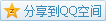# MIT OCW

## 18.02 Multivariable Calculus

### Equations of Lines

We have known that a line can be treated as the intersection of two planes, so it's okay to describe a line by two equations, but not that natural.
Here's another way, a line can be treated as the trajectory of a moving point.

e.g. Line through $Q_0(-1,2,2),Q_1(1,3,-1)$
Solution Select a point $Q(x,y,z)$ on the plane, and we should have $\mathbf{Q_0Q}=t\mathbf{Q_0Q_1}=(2,1,-3)$, for some $t$. Let's consider $t$ as a parameter and expand the formula that is $$\begin{bmatrix}x+1\\y-2\\z-2\end{bmatrix}=t\begin{bmatrix}2\\1\\-3\end{bmatrix}.$$
We can write $x,y,z$ as functions with respect to $t$ as the following \left\{\begin{aligned}x(t)&=&-1&+2t\\y(t)&=&2&+t\\z(t)&=&2&-3t\end{aligned}\right..

#### Intersection with a Plane

e.g. What's $Q_0(-1,2,2)$ and $Q_1(1,3,-1)$ like relative to $x+2y+4z=7$?
Same side / Opposite sides / One is on the plane.
Solution The plane $x+2y+4z=7$ is actually dividing the whole space into two regions where one fulfills $x+2y+4z>7$ and the other $x+2y+4z<7$. We just plugin these two points into the plane equation and check the equality.
For $Q_0$, $-1+2\times 2+4\times 2=11>7$; and for $Q_1$, $1+2\times 3+4\times(-1)=3<7$, so neither of them is on the plane and they are on the opposite sides.
To get the intersection, we just plug the parametric equation of the line into the plane equation that is $$x(t)+2y(t)+4z(t)=7,$$
after solving, we can get $t=\frac12$, and indicating the intersection is $(0,\frac25,\frac12)$.

### Parametric Equations of Curves

#### Cycloid

The whole process is just a trick in geometry, the parametric equation for a cycloid with radius $a$ is
\left\{ \begin{aligned} x(\theta)&=a(\theta-\sin(\theta))\\ y(\theta)&=a(1-\cos(\theta)) \end{aligned} \right..
Let's observe the edge like the place near $\theta=0,2\pi,\cdots$. Without loss of generality, take $\theta=0$ as an example.
According to Taylor's theorem, $\sin(\theta)\sim\theta-\frac{\theta^3}{3!}$ and $\cos\theta\sim1-\frac{\theta^2}{2!}$, consider the slope near $\theta=0$ that is $\frac{y(t)}{x(t)}=\frac{1-\cos(\theta)}{\theta-\sin(\theta)}~\frac{\frac{\theta^2}{2!}}{\frac{\theta^3}{3!}}=\frac{3}{\theta}\to\infty$, indicating that the behavior near $\theta=0$ is to toggle some motion, which is obvious to be understood when we imagine a wheel rolling on the ground.